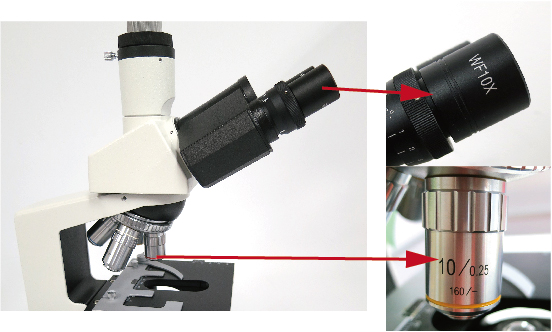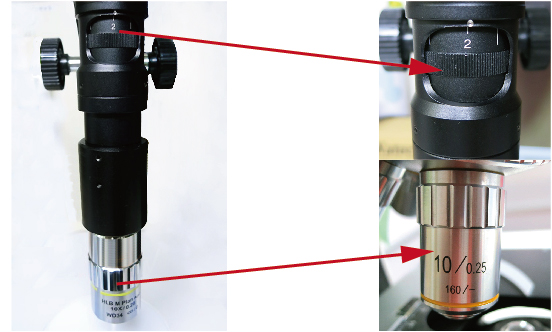# What’s the difference between optical microscope magnification and digital microscope magnification?

The magnification of the optical microscope and the magnification of digital microscope are very different.

The magnification of optical microscope is absolute magnification, even if the manufacturer differs, if the magnification is the same, it will be in the same field of view. (It is an absolute magnification.)

However, for digital microscope, the field of view will be very different depending on the manufacturer even at the same magnification. (Relative magnification.)

Optical Microscope magnification

The magnification of the microscope is calculated by the following formula.

Optical magnification = (magnification of the objective lens) × (magnification of the eyepiece) Magnification of the microscope = optical magnification.

(In the case of the microscope of the bottom photograph, the magnification becomes 10 times the magnification of the objective lens × the magnification of the eyepiece 10 times, the optical magnification becomes 100 times.)Even in the case of a microscope, when the camera is attached to microscope, it may be expressed including the monitor magnification.

(Refer to “How to calculate magnification of camera for microscope”.)

Digital Microscope magnification

Taking an example of a microscope with an objective lens, which is easy to understand.

Firstly, the calculation of optical magnification is the same as the microscope.

Optical magnification = (magnification of the objective lens) × (magnification of the lens)

(In the microscope of the below picture, the magnification of the objective lens is 10 × magnification × 2 times the magnification of the lens body, the optical magnification is “20 times.”)However, the monitor magnification is shown here.

Overall magnification = monitor magnification × optical magnification.

If the image sensor size is 1/2 inch and the monitor is 17 inches, the monitor magnification is 54.

(Please refer to “magnification of microscope”.)

Overall magnification = monitor magnification × optical magnification = 54 × 20 = 1080 times.

In conclusion, if the monitor size or image sensor size changes, the magnification will also change.

(Looking at the catalog of each manufacturer, there is a note such as “Equivalent to” ○○ inches monitor ”

< Magnification definition>

Computing is complex, but the way of thinking is simple.

Microscope as shown below with 1mm on the display to 40mm.

This is simply 40 times.